Published

# PIC Wave Player

SD Card pic16f690 FAT32 wave player.

IntermediateFull instructions provided57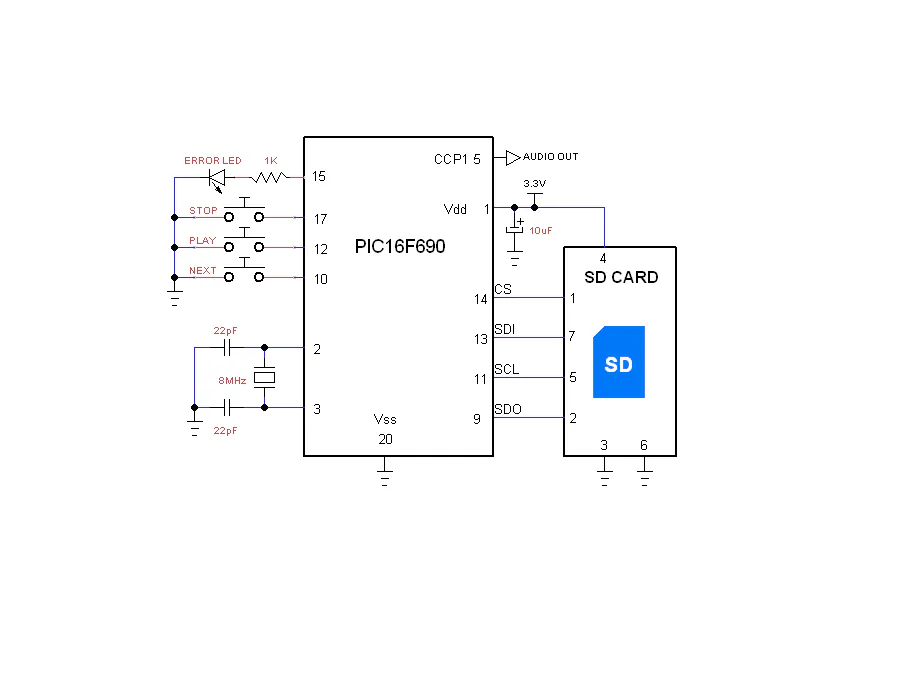## Things used in this project

### Hardware components

 Microchip pic16f690
×1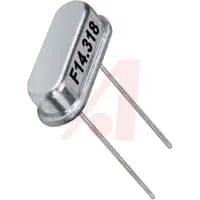8 MHz Crystal
×1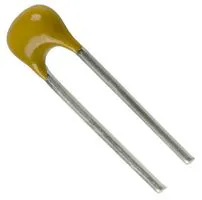Capacitor 22 pF
×1SparkFun Pushbutton switch 12mm
×3LED (generic)
×1Resistor 1k ohm
×1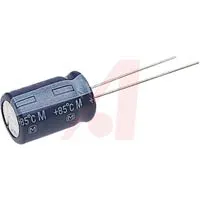Capacitor 10 µF
×1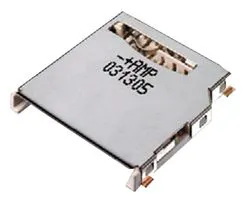Memory Socket, SD Card
×1

## Schematics

### FAT32 player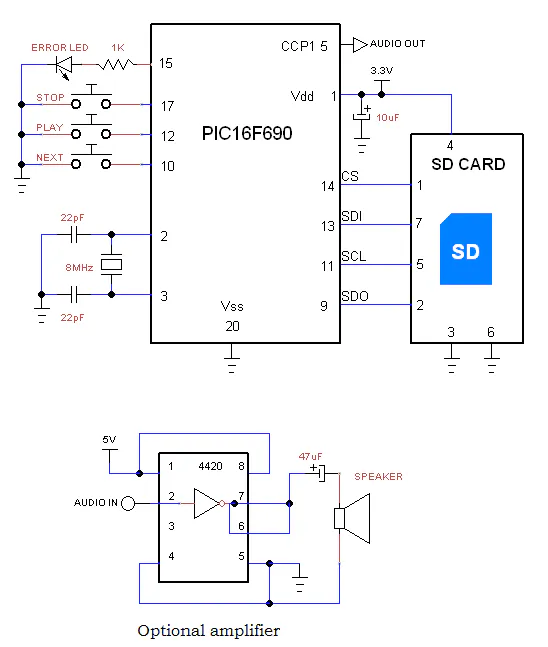## Code

### sd player

C/C++
```//*          SD FAT32 Player      *
//
// Compile with MPLAB Hi Tech or MPLABX XC8.
// pic16f690

#include <htc.h>

#define _XTAL_FREQ 8000000

#define CSsd RC2		//chip select input
#define Stop RA2	//stop pushbutton
#define Play RB5	//play PB
#define Next RB7		//next track PB //RB7
//#define Pause RA1	//pause PB
#define errorLED RC1
#define sdLED RC4	//SD or SDHC optional LED

//prototypes
void main(void);
unsigned char SPI(unsigned char spidata);
char Command(unsigned char frame1, unsigned long adrs, unsigned char frame2 );
void initSD(void);
void fat (void);
void file(unsigned int offset, unsigned char sect);

#if defined(__XC8)
#pragma config FOSC=HS, CP=OFF, CPD=OFF, WDTE=OFF, BOREN=OFF, MCLRE=OFF
#else defined(COMPILER_MPLAB_PICC)
__CONFIG(WDTDIS & UNPROTECT & HS & MCLRDIS & UNPROTECT & BORDIS & PWRTDIS);	//LVPDIS &
#endif

unsigned long loc,BootSector, RootDir, SectorsPerFat, RootDirCluster, DataSector, FileCluster, FileSize;	//
unsigned int BytesPerSector, ReservedSectors, card;	//, RootEntries

unsigned char sdhc=0, SectorsPerCluster, Fats;	//standard sd

void main(void)
{
unsigned char fn=1, sn=0;	//file #, sector#

//OSCCON = 0B1110001;	//8MHz,
// PIC I/O init
ANSEL = 0;
ANSELH = 0;
TRISC = 0b1000000;		//  rc5=CPP1.
TRISA = 0b110;   	// switches
TRISB = 0b10111111;
//pullup on
//RABPU = 0;
#if defined(__XC8)
nRABPU = 0;
#else defined(COMPILER_MPLAB_PICC)
RABPU = 0;
#endif
//WPUA1 = 1;
WPUA2 = 1;
WPUB5 = 1;
WPUB7 = 1;

//ANS8 = 1;	//analogue chan 8
//RecLED = 0;
errorLED = 0;
sdLED = 0;

//analogue init
CCP1CON = 0B1100;	//PWM mode
PR2 = 63;	//33KHz PWM
T2CON = 0B100;	//prescale 1, post scale 1, timer2 on
//timer1
T0CS=0;			//timer0 Internal clock
PSA=1;			//Prescaler is assigned to the WDT

//SPI init
SSPCON = 0B110010;	//low speed osc/64(125kHz),enabled,clock idle=H
CSsd = 1; 		// disable SD

initSD();
if(sdhc){card = 1;}else{card=512;}	//SD or SDHC
fat();
if(BytesPerSector!=512){errorLED=1;}

while(1){
if(!Play){
while(Stop){
file(fn*32+20,sn);		//32 bytes per file descriptor at offset of 20
if(FileCluster){	//cluster reads 0 is end of files entries
loc=(1 + (DataSector) + (unsigned long)(FileCluster-2) * SectorsPerCluster) * card ;
//__delay_ms(250);
while(!Next){}
if(Stop){++fn;}
if(fn>15){fn=0;++sn;}
}else{fn=1;sn=0;}
}
}
}
}

void file(unsigned int offset, unsigned char sect)	//find files
{
unsigned int r,i;
unsigned char fc, fs; //

CSsd = 0;
r = Command(17,(RootDir+sect)*card,0xFF);		//read boot-sector for info from file entry
if(r != 0)errorLED = 1;			//if command failed

while(SPI(0xFF) != 0xFe);	// wait for first byte
for(i=0;i<512;i++){
if(i==offset){fc=SPI(0xFF);}
else if(i==offset+1){fc=SPI(0xFF);}
else if(i==offset+6){fc=SPI(0xFF);}
else if(i==offset+7){fc=SPI(0xFF);}

else if(i==offset+8){fs=SPI(0xFF);}
else if(i==offset+9){fs=SPI(0xFF);}
else if(i==offset+10){fs=SPI(0xFF);}
else if(i==offset+11){fs=SPI(0xFF);}
else{SPI(0xFF);}

}
SPI(0xFF);
CSsd = 1;SPI(0xFF);
FileCluster = fc | ( (unsigned long)fc << 8 ) | ( (unsigned long)fc << 16 ) | ( (unsigned long)fc << 24 );
FileSize = fs | ( (unsigned long)fs << 8 ) | ( (unsigned long)fs << 16 ) | ( (unsigned long)fs << 24 );
FileSize = FileSize/512+1;		//file size in sectors
}

{
unsigned int i,r;
unsigned char data;

CSsd = 0;
if(r != 0)errorLED = 1;			//if command failed

while(Stop && Next && FileSize--)
{
while(SPI(0xFF) != 0xFE);	// wait for first byte
for(i=0;i<512;i++){
while(!T0IF){}
TMR0=165;		//(256-91) 91 counts to get 22KHz, play speed
T0IF = 0;
data = SPI(0xFF);
DC1B1 = data & 2;	//shift byte to get the required PWM duty cycle
DC1B0 = data & 1;
CCPR1L = (data >> 2);
}
SPI(0xFF);
}
Command(12,0x00,0xFF);	//stop transmit
SPI(0xFF);
SPI(0xFF);
CSsd = 1;SPI(0xFF);
}

void fat (void)
{
unsigned int r,i;
unsigned char pfs,bps1,bps2,rs1,rs2,spf,rdc; //pfs=partition first sector ,de1,de2,spf1,d

CSsd = 0;
if(r != 0)errorLED = 1;			//if command failed

while(SPI(0xFF) != 0xFe);	// wait for first byte
for(i=0;i<512;i++){
if(i==454){pfs=SPI(0xFF);}	//pfs=partition first sector
else if(i==455){pfs=SPI(0xFF);}
else if(i==456){pfs=SPI(0xFF);}
else if(i==457){pfs=SPI(0xFF);}
else{SPI(0xFF);}

}
SPI(0xFF);
CSsd = 1;SPI(0xFF);
//convert 4 bytes to long int
BootSector = pfs | ( (unsigned long)pfs << 8 ) | ( (unsigned long)pfs << 16 ) | ( (unsigned long)pfs << 24 );

CSsd = 0;
if(r != 0)errorLED = 1;			//if command failed

while(SPI(0xFF) != 0xFe);	// wait for first byte
for(i=0;i<512;i++){

if(i==11){bps1=SPI(0xFF);} //bytes per sector
else if(i==12){bps2=SPI(0xFF);}
else if(i==13){SectorsPerCluster=SPI(0xFF);}
else if(i==14){rs1=SPI(0xFF);}
else if(i==15){rs2=SPI(0xFF);}
else if(i==16){Fats=SPI(0xFF);}	//number of FATs
else if(i==36){spf=SPI(0xFF);}
else if(i==37){spf=SPI(0xFF);}
else if(i==38){spf=SPI(0xFF);}
else if(i==39){spf=SPI(0xFF);}
else if(i==44){rdc=SPI(0xFF);}
else if(i==45){rdc=SPI(0xFF);}
else if(i==46){rdc=SPI(0xFF);}
else if(i==47){rdc=SPI(0xFF);}
else{SPI(0xFF);}

}
SPI(0xFF);
CSsd = 1;SPI(0xFF);

BytesPerSector = bps1 | ( (unsigned int)bps2 << 8 );
ReservedSectors = rs1 | ( (unsigned int)rs2 << 8 );	//from partition start to first FAT
RootDirCluster = rdc | ( (unsigned long)rdc << 8 ) | ( (unsigned long)rdc << 16 ) | ( (unsigned long)rdc << 24 );
SectorsPerFat = spf | ( (unsigned long)spf << 8 ) | ( (unsigned long)spf << 16 ) | ( (unsigned long)spf << 24 );
DataSector = BootSector + (unsigned long)Fats * (unsigned long)SectorsPerFat + (unsigned long)ReservedSectors;	// + 1
RootDir = (RootDirCluster -2) * (unsigned long)SectorsPerCluster + DataSector;
}

unsigned char SPI(unsigned char spidata)		// send character over SPI
{
SSPBUF = spidata;			// load character
while (!BF);		// sent
}

char Command(unsigned char frame1, unsigned long adrs, unsigned char frame2 )
{
unsigned char i, res;

//SPI(0xFF);
SPI((frame1 | 0x40) & 0x7F);	//first 2 bits are 01
SPI(frame2 | 1);				//CRC and last bit 1

for(i=0;i<10;i++)	// wait for received character
{
res = SPI(0xFF);
if(res != 0xFF)break;
}
return res;
}

void initSD(void)
{
unsigned char i,r;

CSsd=1;
for(i=0; i < 10; i++)SPI(0xFF);		// min 74 clocks
CSsd=0;			// Enabled for SPI mode

//if (Command(0x00,0,0x95) !=1) errorLED = 1;	//start SPI mode
i=100;	//try enter idle state for up to 100 times
while(Command(0x00,0,0x95) !=1 && i!=0)
{
CSsd=1;
SPI(0xFF);
CSsd=0;
i--;
}
if(i==0)	errorLED = 1;	//idle failed

if (Command(8,0x01AA,0x87)==1){					//check card is 3.3V
r=SPI(0xFF); r=SPI(0xFF); r=SPI(0xFF); r=SPI(0xFF);		//rest of R7
if ( r == 0x01 && r == 0xAA ){ 		//Vdd OK (3.3V)

//Command(59,0,0xFF);		//CRC off
Command(55,0,0xFF);
while(Command(41,0x40000000,0xFF)){Command(55,0,0xFF);} 	//ACMD41 with HCSsd bit
}
}else{errorLED = 1;}

if (Command(58,0,0xFF)==0){		//read CCSsd in the OCR - SD or SDHC
r=SPI(0xFF); r=SPI(0xFF); r=SPI(0xFF); r=SPI(0xFF);		//rest of R3
sdhc=r & 0x40;
if(r & 0x40)sdLED=1;
}

SSPM1 = 0;	// full speed 2MHz
CSsd = 1;SPI(0xFF);

}
```